# Lecture_4_-_Data_Management_using_Statistics(3).pptx

5 de Nov de 2022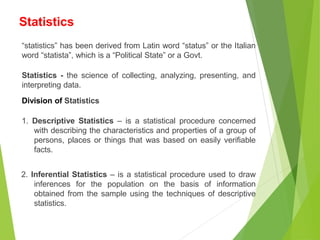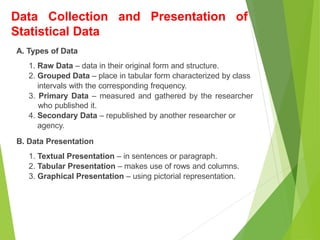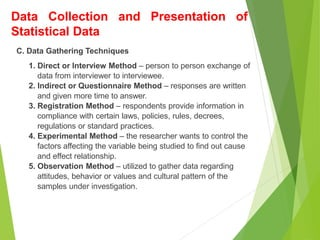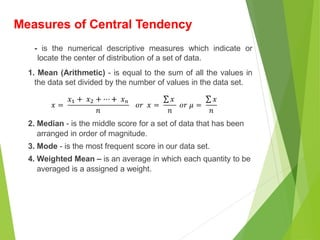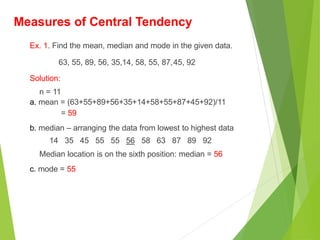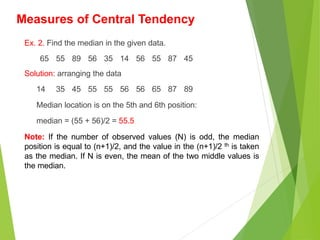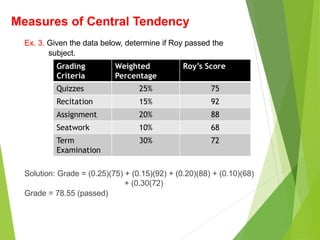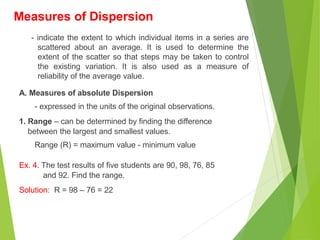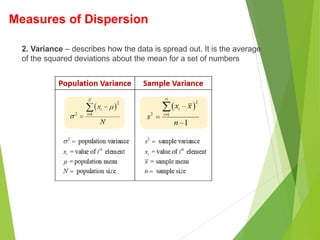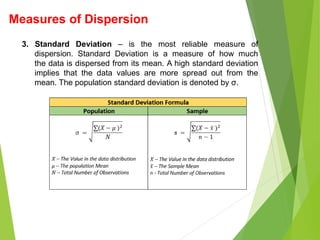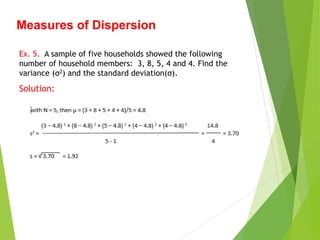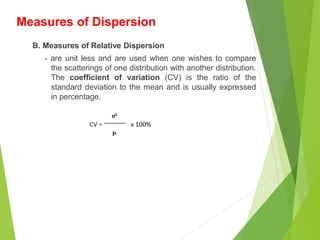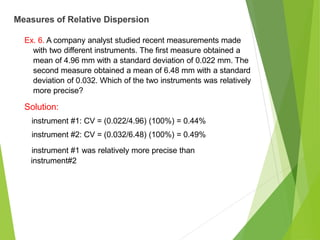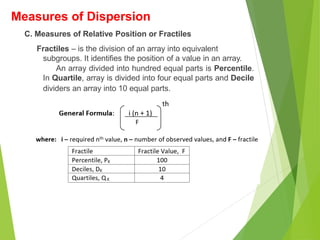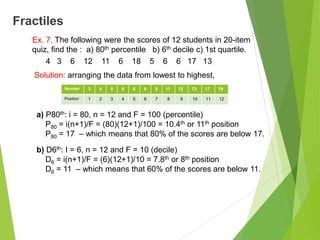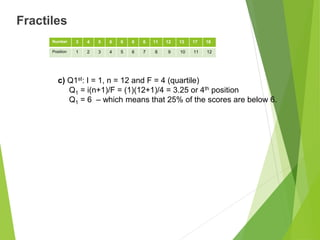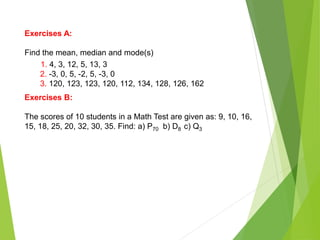1 de 18

### Lecture_4_-_Data_Management_using_Statistics(3).pptx

• 1. Data Management using Statistics
• 2. “statistics” has been derived from Latin word “status” or the Italian word “statista”, which is a “Political State” or a Govt. Statistics - the science of collecting, analyzing, presenting, and interpreting data. Division of Statistics 1. Descriptive Statistics – is a statistical procedure concerned with describing the characteristics and properties of a group of persons, places or things that was based on easily verifiable facts. 2. Inferential Statistics – is a statistical procedure used to draw inferences for the population on the basis of information obtained from the sample using the techniques of descriptive statistics. Statistics
• 3. Data Collection and Presentation of Statistical Data A. Types of Data 1. Raw Data – data in their original form and structure. 2. Grouped Data – place in tabular form characterized by class intervals with the corresponding frequency. 3. Primary Data – measured and gathered by the researcher who published it. 4. Secondary Data – republished by another researcher or agency. B. Data Presentation 1. Textual Presentation – in sentences or paragraph. 2. Tabular Presentation – makes use of rows and columns. 3. Graphical Presentation – using pictorial representation.
• 4. C. Data Gathering Techniques 1. Direct or Interview Method – person to person exchange of data from interviewer to interviewee. 2. Indirect or Questionnaire Method – responses are written and given more time to answer. 3. Registration Method – respondents provide information in compliance with certain laws, policies, rules, decrees, regulations or standard practices. 4. Experimental Method – the researcher wants to control the factors affecting the variable being studied to find out cause and effect relationship. 5. Observation Method – utilized to gather data regarding attitudes, behavior or values and cultural pattern of the samples under investigation. Data Collection and Presentation of Statistical Data
• 5. - is the numerical descriptive measures which indicate or locate the center of distribution of a set of data. 1. Mean (Arithmetic) - is equal to the sum of all the values in the data set divided by the number of values in the data set. 2. Median - is the middle score for a set of data that has been arranged in order of magnitude. 3. Mode - is the most frequent score in our data set. 4. Weighted Mean – is an average in which each quantity to be averaged is a assigned a weight. Measures of Central Tendency
• 6. Ex. 1. Find the mean, median and mode in the given data. 63, 55, 89, 56, 35,14, 58, 55, 87,45, 92 Solution: n = 11 a. mean = (63+55+89+56+35+14+58+55+87+45+92)/11 = 59 b. median – arranging the data from lowest to highest data 14 35 45 55 55 56 58 63 87 89 92 Median location is on the sixth position: median = 56 c. mode = 55 Measures of Central Tendency
• 7. Ex. 2. Find the median in the given data. 65 55 89 56 35 14 56 55 87 45 Solution: arranging the data 14 35 45 55 55 56 56 65 87 89 Median location is on the 5th and 6th position: median = (55 + 56)/2 = 55.5 Note: If the number of observed values (N) is odd, the median position is equal to (n+1)/2, and the value in the (n+1)/2 th is taken as the median. If N is even, the mean of the two middle values is the median. Measures of Central Tendency
• 8. Ex. 3. Given the data below, determine if Roy passed the subject. Solution: Grade = (0.25)(75) + (0.15)(92) + (0.20)(88) + (0.10)(68) + (0.30(72) Grade = 78.55 (passed) Measures of Central Tendency Grading Criteria Weighted Percentage Roy’s Score Quizzes 25% 75 Recitation 15% 92 Assignment 20% 88 Seatwork 10% 68 Term Examination 30% 72
• 9. - indicate the extent to which individual items in a series are scattered about an average. It is used to determine the extent of the scatter so that steps may be taken to control the existing variation. It is also used as a measure of reliability of the average value. A. Measures of absolute Dispersion - expressed in the units of the original observations. 1. Range – can be determined by finding the difference between the largest and smallest values. Range (R) = maximum value - minimum value Ex. 4. The test results of five students are 90, 98, 76, 85 and 92. Find the range. Solution: R = 98 – 76 = 22 Measures of Dispersion
• 10. 2. Variance – describes how the data is spread out. It is the average of the squared deviations about the mean for a set of numbers Measures of Dispersion
• 11. 3. Standard Deviation – is the most reliable measure of dispersion. Standard Deviation is a measure of how much the data is dispersed from its mean. A high standard deviation implies that the data values are more spread out from the mean. The population standard deviation is denoted by σ. Measures of Dispersion
• 12. Measures of Dispersion Ex. 5. A sample of five households showed the following number of household members: 3, 8, 5, 4 and 4. Find the variance (σ2) and the standard deviation(σ). Solution:
• 13. B. Measures of Relative Dispersion - are unit less and are used when one wishes to compare the scatterings of one distribution with another distribution. The coefficient of variation (CV) is the ratio of the standard deviation to the mean and is usually expressed in percentage. Measures of Dispersion
• 14. Ex. 6. A company analyst studied recent measurements made with two different instruments. The first measure obtained a mean of 4.96 mm with a standard deviation of 0.022 mm. The second measure obtained a mean of 6.48 mm with a standard deviation of 0.032. Which of the two instruments was relatively more precise? Solution: instrument #1: CV = (0.022/4.96) (100%) = 0.44% instrument #2: CV = (0.032/6.48) (100%) = 0.49% instrument #1 was relatively more precise than instrument#2 Measures of Relative Dispersion
• 15. C. Measures of Relative Position or Fractiles Fractiles – is the division of an array into equivalent subgroups. It identifies the position of a value in an array. An array divided into hundred equal parts is Percentile. In Quartile, array is divided into four equal parts and Decile dividers an array into 10 equal parts. Measures of Dispersion
• 16. Fractiles Ex. 7. The following were the scores of 12 students in 20-item quiz, find the : a) 80th percentile b) 6th decile c) 1st quartile. 4 3 6 12 11 6 18 5 6 6 17 13 Solution: arranging the data from lowest to highest, a) P80th: i = 80, n = 12 and F = 100 (percentile) P80 = i(n+1)/F = (80)(12+1)/100 = 10.4th or 11th position P80 = 17 – which means that 80% of the scores are below 17. b) D6th: I = 6, n = 12 and F = 10 (decile) D6 = i(n+1)/F = (6)(12+1)/10 = 7.8th or 8th position D6 = 11 – which means that 60% of the scores are below 11. Number 3 4 5 6 6 6 6 11 12 13 17 18 Position 1 2 3 4 5 6 7 8 9 10 11 12
• 17. Fractiles c) Q1st: I = 1, n = 12 and F = 4 (quartile) Q1 = i(n+1)/F = (1)(12+1)/4 = 3.25 or 4th position Q1 = 6 – which means that 25% of the scores are below 6. Number 3 4 5 6 6 6 6 11 12 13 17 18 Position 1 2 3 4 5 6 7 8 9 10 11 12
• 18. Exercises A: Find the mean, median and mode(s) 1. 4, 3, 12, 5, 13, 3 2. -3, 0, 5, -2, 5, -3, 0 3. 120, 123, 123, 120, 112, 134, 128, 126, 162 Exercises B: The scores of 10 students in a Math Test are given as: 9, 10, 16, 15, 18, 25, 20, 32, 30, 35. Find: a) P70 b) D8 c) Q3